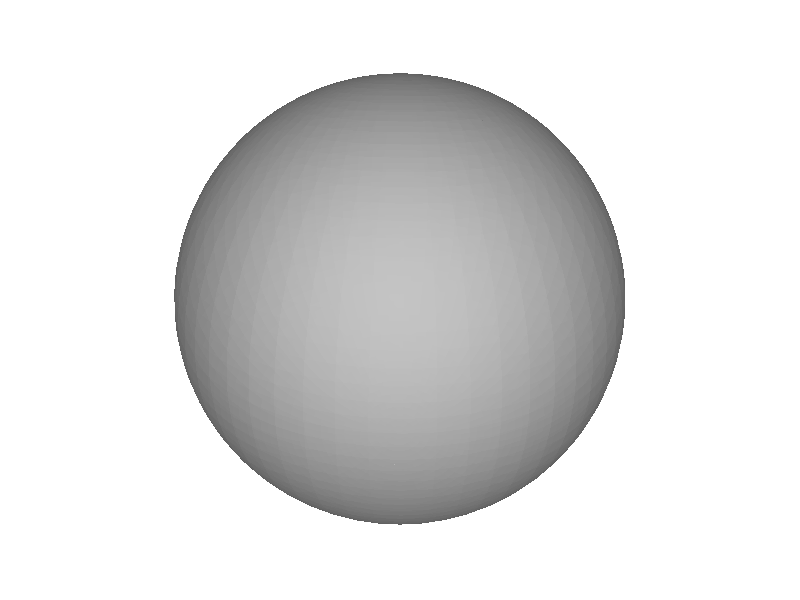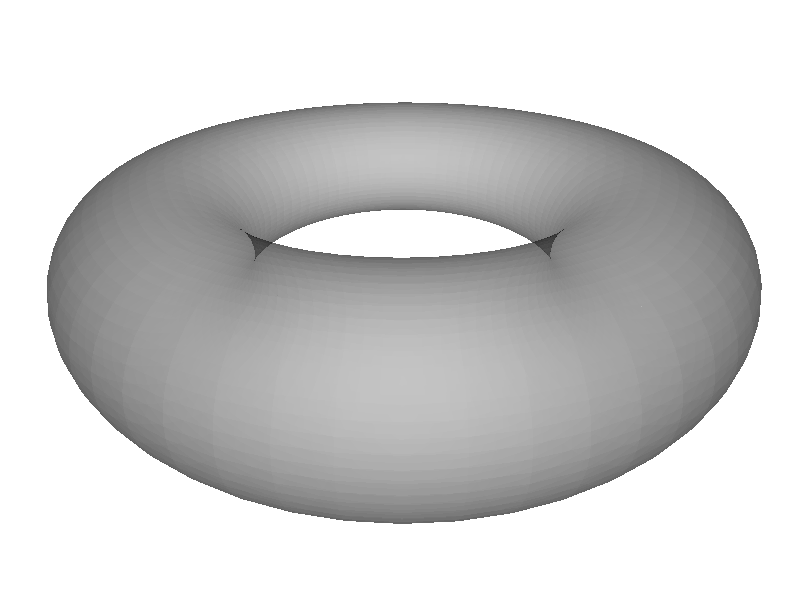Tour

Surface Models

# Building a Geometry¶

## The Mesh Class¶

class chroma.geometry.Mesh(vertices, triangles, remove_duplicate_vertices=False)

Triangle mesh object.

assemble(key=slice(None, None, None), group=True)

Return an assembled triangle mesh; i.e. return the vertex positions of every triangle. If group is True, the array returned will have an extra axis denoting the triangle number; i.e. if the mesh contains N triangles, the returned array will have the shape (N,3,3). If group is False, return just the vertex positions without any grouping; in this case the grouping is implied (i.e. elements [0:3] are the first triangle, [3:6] the second, and so on.

The key argument is a slice object if you just want to assemble a certain range of the triangles, i.e. to get the assembled mesh for triangles 3 through 6, key = slice(3,7).

get_bounds()

Return the lower and upper bounds for the mesh as a tuple.

get_triangle_centers()

Returns the x,y,z coordinate of the center of each triangle.

md5()

Return the MD5 hash of the vertices and triangles in this mesh as a hexidecimal string.

remove_duplicate_vertices()

Remove any duplicate vertices in the mesh.

remove_null_triangles()

Remove any zero-area triangles from a mesh.

Returns the mask of retained triangles, which may be applied to the material, surface, etc., arrays of an associated Solid.

## The Solid Class¶

class chroma.geometry.Solid(mesh, material1=None, material2=None, surface=None, color=872415231)

Solid object attaches materials, surfaces, and colors to each triangle in a Mesh object.

weld(other, shared_triangle_surface=None, shared_triangle_color=None)

Merge this solid with another at any identical triangles.

Triangles that are common to both solids will be reduced to one, with the surface and color properties of this one unless otherwise specified with shared_triangle_surface and/or shared_triangle_color.

Note that this is NOT a boolean union!

## The Material Class¶

class chroma.geometry.Material(name='none')

Material optical properties.

## The Geometry Class¶

class chroma.geometry.Geometry(detector_material=None)

Geometry object.

Add the solid solid to the geometry. When building the final triangle mesh, solid will be placed by rotating it with the rotation matrix rotation and displacing it by the vector displacement.

flatten()

Create the flat list of triangles (and triangle properties) from the list of solids in this geometry.

This does not build the BVH! If you want to use the geometry for rendering or simulation, you should call build() instead.

## Importing from STL¶

chroma.mesh_from_stl(filename)

Returns a chroma.geometry.Mesh from an STL file.

## Mesh Modelling Tools¶

chroma.make.linear_extrude(x1, y1, height, x2=None, y2=None, center=None, endcaps=True)

Return the solid mesh formed by linearly extruding the polygon formed by the x and y points x1 and y1 by a distance height. If x2 and y2 are given extrude by connecting the points x1 and y1 to x2 and y2; this allows the creation of tapered solids. If endcaps is False, then the triangles on the endcaps will be left off, and the mesh will not be closed.

Note

The path traced by the points x and y should go counter-clockwise, otherwise the mesh will be inside out.

Example:
```>>> # create a hexagon prism
>>> angles = np.linspace(0, 2*np.pi, 6, endpoint=False)
>>> m = linear_extrude(np.cos(angles), np.sin(angles), 2.0)
```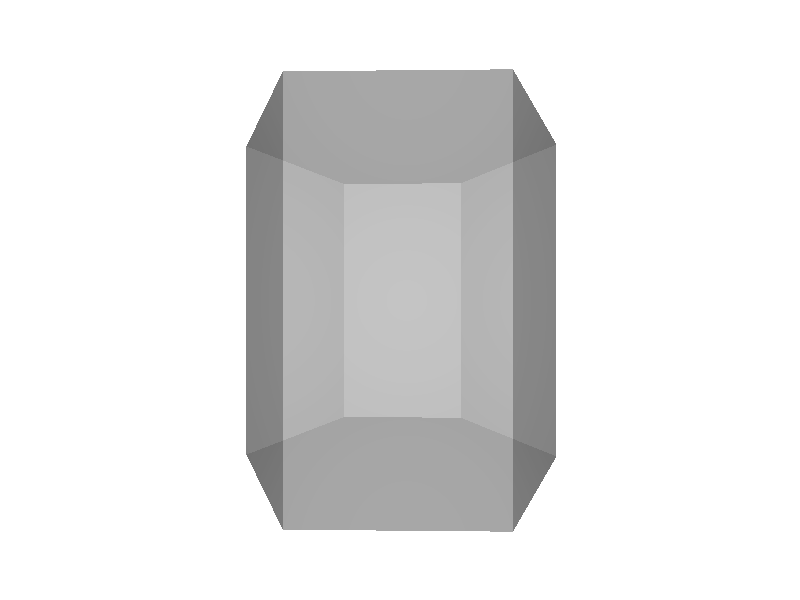chroma.make.rotate_extrude(x, y, nsteps=64)

Return the solid mesh formed by extruding the profile defined by the x and y points x and y around the y axis.

Note

The path traced by the points x and y should go counter-clockwise, otherwise the mesh will be inside out.

Example:
```>>> # create a bipyramid
>>> m = rotate_extrude([0,1,0], [-1,0,1], nsteps=4)
```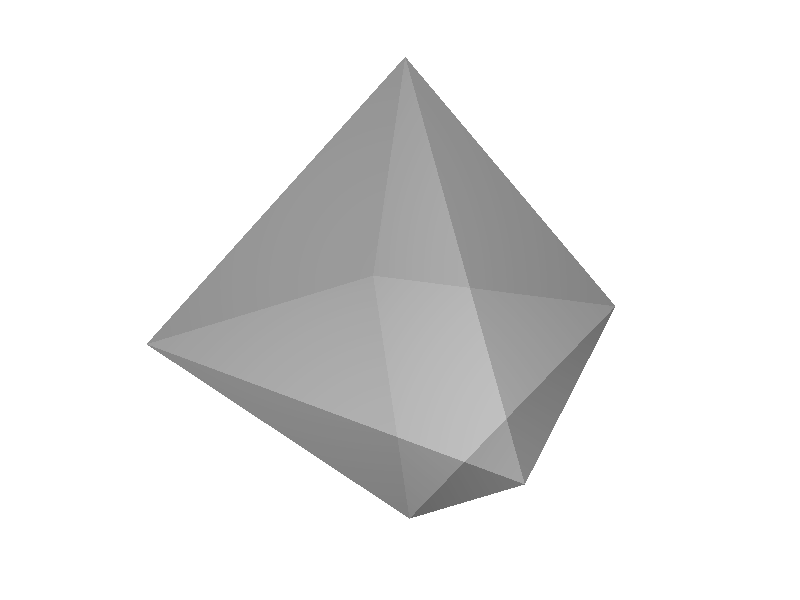chroma.make.box(dx, dy, dz, center=(0, 0, 0))

Return a box with linear dimensions dx, dy, and dz.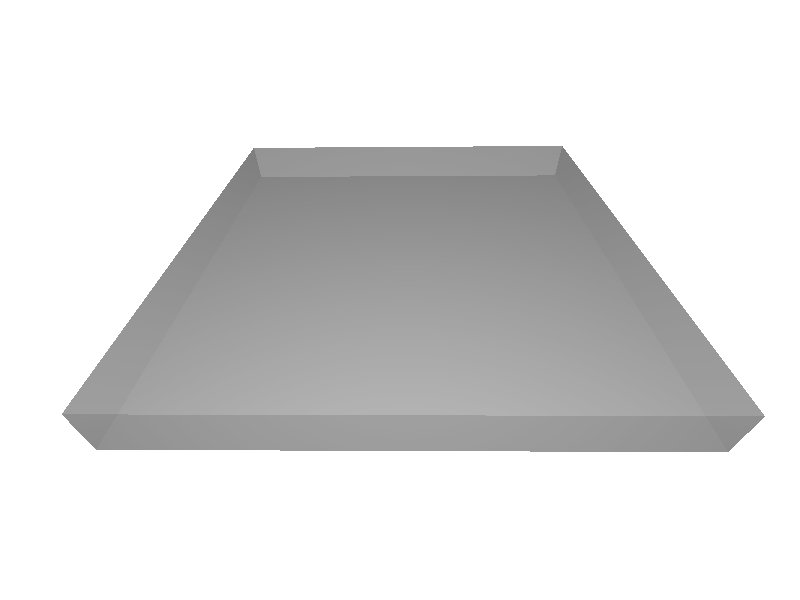chroma.make.cube(size, height=None, center=(0, 0, 0))

Return a cube mesh whose sides have length size.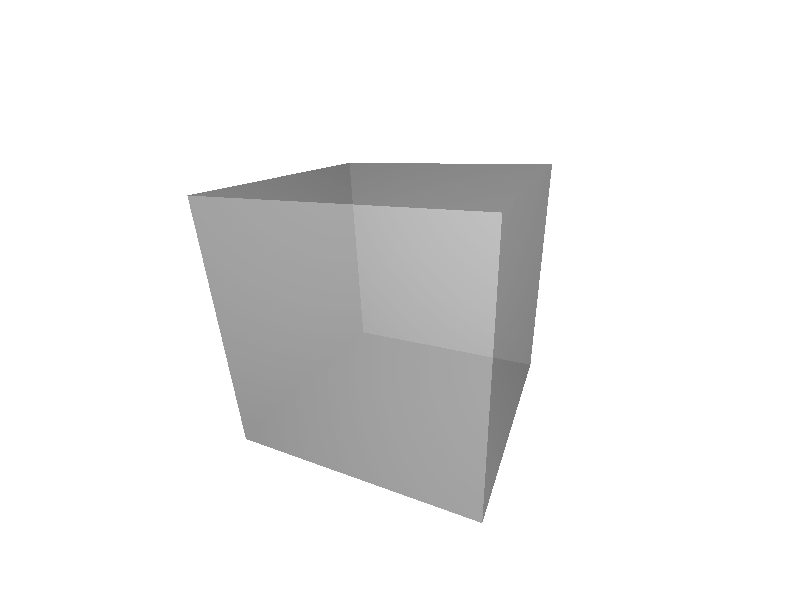Return a cylinder mesh with a radius of length radius, and a height of length height. If radius2 is specified, return a cone shaped cylinder with bottom radius radius, and top radius radius2.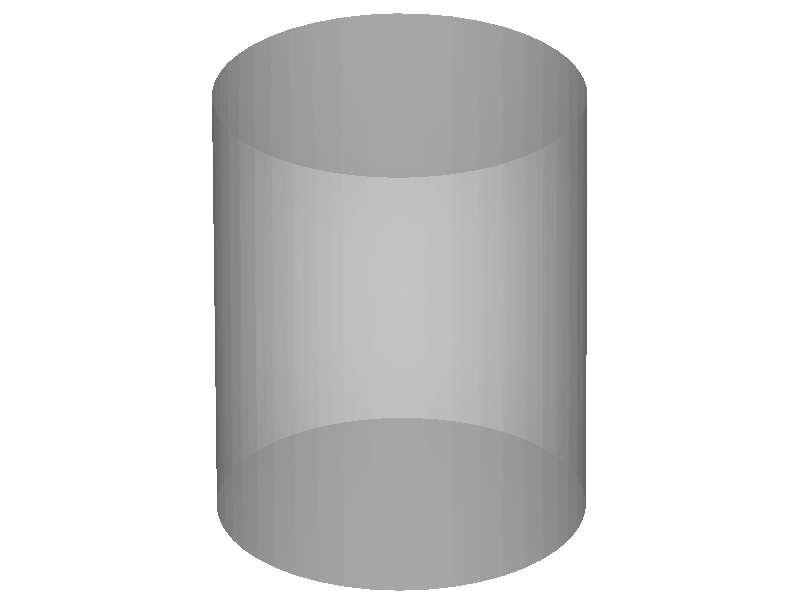chroma.make.segmented_cylinder(radius, height, nsteps=64, nsegments=100)

Return a cylinder mesh segmented into nsegments points along its profile.